# Extending Our Senses: Indirect Measurement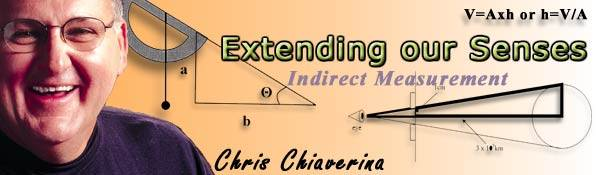Everything we experience comes to us through our five senses—sight, hearing, touch, smell and taste. While our senses are truly amazing, most of what goes on around us occurs unnoticed. Since we can only see a small range of the electromagnetic spectrum as visible light, we can be in the vicinity of a radio-transmitting tower radiating 50,000 watts of power and be totally unaware of its presence. The fluttering of a hummingbird wing and changes in mountain ranges are undetectable to the average human. Extreme distances, both short and long, are equally elusive. We can see the dot above an "i", but cannot see a grain of pollen. At the other outer limits of length, we can only imagine what a light year is.

Scientific research includes the study of subatomic particles as well as the mind-boggling distances that exist between the earth and neighboring stars and nebulae. This great breadth of investigation involves extending our senses and developing new ways of "seeing".

Scientific instruments that enable us to overcome our sensory limitations have been, and continue to be, essential to the progress of science. The microscope and the telescope provide mankind with windows to two previously unseen worlds. The stroboscope has enabled us to "freeze" motion. X-rays have provided a non-invasive way of probing the body. Radio telescopes enable us to extend our grasp to the far reaches of space. Cloud and bubble chambers allow us to study events occurring on the subatomic scale.

In a similar way, the following experiments will allow your students to extend their senses and make measurements they never dreamed possible. They will determine the size of a molecule, time events that occur in an instant, and measure dimensions on an astronomical scale. In the process, they will learn how scientists make observations and measurements in the invisible world.

# 1. Measuring New Heights

Key Concept: Students are asked to indirectly measure the height of an object much larger than their available measuring instrument.

Give the students instructions below, and turn them loose! Give them time to plan in the classroom before going out as a group to make measurements. Give as little advice as possible. Their methods (and the results) may vary a lot, and that's okay!

The challenge: Determine the height of a tall object on the school grounds such as a flagpole, a chimney, or other structure identified by your teacher. You will only be allowed the use of a meter stick. You may think that you don't have the knowledge to make such a measurement with so little equipment, but you would be wrong!

Before leaving the classroom, do some brainstorming with members of your group. You will be surprised to learn that you have the ability to measure such a tall object indirectly. Each group will be asked to share not only their value for the height of the object, but more importantly, their method. So be ready!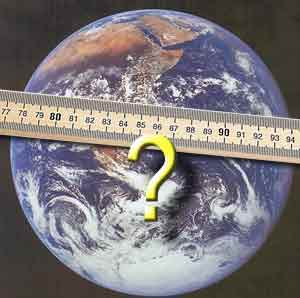### 2. Blast Off!

Key Concept: The measurement method known as triangulation can be used to indirectly determine the heights of tall structures or the altitudes of projectiles.

Demonstrate the calculations for the class before assigning each group a height or altitude to measure. Trigonometry is involved, but students really only need to do some basic algebra to grasp this concept. You can use triangulation to find the height of buildings or as part of other labs and activities like Bottle Rockets.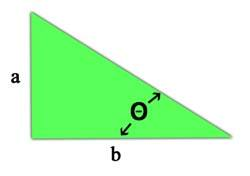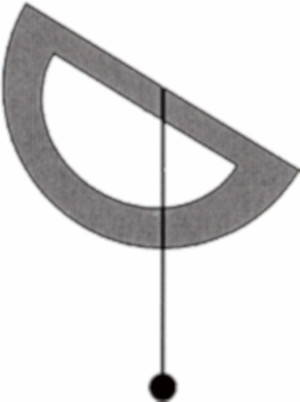Trigonometry provides an easy way to determine the heights of structures or even the altitude of a toy rocket. Trigonometry deals with ratios of the lengths of pairs of the sides of a right triangle. You may have heard of the sine, cosine, and tangent. Scary sounding? Perhaps, but don't worry, they're all just ratios. To make things easier, we'll only consider the tangent.

The tangent of an angle (Θ, "theta") is the ratio of the length of the side opposite the angle to the length of the side adjacent to the angle. In other words, it's the ratio of side a to side b. This ratio increases as the angle of inclination Θ increases. The tangent for angles between 0 and 90 degrees may found in a table or calculator.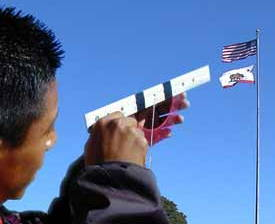Suppose you fire a rocket into the air and wish to know its altitude. If you know the distance from you to the launch point (b) and the angle of inclination (Θ), you can find the rocket's altitude (a) becausetangent Θ = a/b
or
a = b tangent Θ(Hint: You can look up the tangent of any angle from 0 to 90 in a table or by using your scientific calculator.)Voila! The tangent makes the indirect measurement of heights a snap.

To actually carry out a measurement of a rocket's altitude, you will need a protractor (an instrument used for measuring angles), a string with a small weight on the end (also known as a plum bob), a meter stick, and a tangent table or calculator.

After tying a weight to the end of a string, attach the string to the center of the protractor (see fig. 2). This device will enable you to determine the angle of inclination. Now all you need is the baseline b, the distance between the launch pad and where you stand when you sight on the rocket.

When the rocket reaches its maximum altitude, view the rocket along the edge of the protractor. Have your lab partner observe the angle indicated by the string. Because the protractor is inverted, this angle must be subtracted from 900 to obtain the angle of inclination. To find the altitude of the rocket, simply multiply the tangent of the angle of incidence by the length of the baseline.

# 3. Measuring the Moon

Key Concept: Students will use the concept of similar triangles to indirectly measure the diameter of the moon.

This activity can be done in the classroom, if the moon happens to be visible from your windows, or it can be done at home by each student.Student Instructions:
You may find this hard to believe, but you can measure the diameter of the moon from the comfort of your home. The equipment needed includes an index card, a pin, two strips of opaque tape (masking or electrical tape works well), and a centimeter ruler.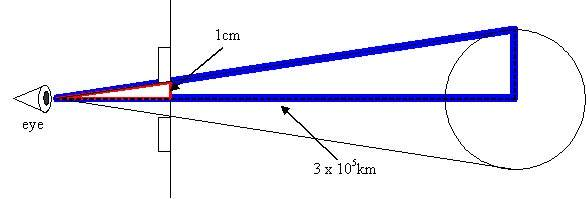Oh, and one other thing, you'll need to know that the moon is 3 x 105 km from earth.

When the moon is full, place the two strips of tape 2cm apart on a windowpane facing the moon. After making a pinhole in the index card, observe the moon through the pinhole and two strips of tape. Back away from the window until the moon appears to just fill the space between the two strips of tape. Measure the distance from the card to the window. Using the proportionality of sides that exists for similar triangles (see figure above), calculate the diameter of the moon.

# 4. Measuring Molecular Monolayers

Key Concept: Students will use the volume of a large number of items and the area covered by a single layer of those items to indirectly find the diameter of a single item.

As with any lab using chemicals and glassware, this lab requires appropriate safety measures, such as goggles. The final result for the height of an oleic acid molecule might not be very accurate, but the exercise is still worthwhile. When students are able to measure something that they cannot see, they understand a bit more about how scientists work.

Suppose you wanted to find the diameter of a BB, but didn't have an instrument, such as a micrometer, suited for the job. What could you do?

One way to obtain the diameter of a single BB requires the use of many BB's. Begin by placing a large number of BB's in a graduated cylinder. Record the total volume of BB's. (In carrying out this measurement, you are making an assumption. Do you know what it is?)

Now spread the BB's out in a circular pattern on a table. This results in a monolayer, a cylindrical volume whose depth is a single BB. Measure the diameter of this circle. Because you may have difficulty making a perfect circle, make this measurement a number of times and find the average diameter. Divide the diameter by two, and use this radius to find the area of the circle.

The diameter of one BB is the same as the height of the very flat cylinder you just made. We can use the area of the circle and the total volume of BB's (measured earlier) to find the height. The volume of a cylinder is the area of its base times the height.

V = A x h
or
h = V/A

Note: 1 mL = 1 cm3.

Believe it or not, you can estimate the size of a single molecule using a similar approach. This time however, you will be dealing with a monolayer of molecules rather than a monolayer of BBs. To perform the experiment you'll need a pizza pan, some chalk dust, an eyedropper, a 10-ml graduated cylinder, and oleic acid solution. The oleic acid solution is prepared by adding 5-ml oleic acid to 995-ml ethanol.

After filling the pizza pan with water, spread chalk dust over the surface of the water. Easy does it, for too much powder will hinder the spread of the oleic acid. Using an eyedropper, carefully add just one drop of the oleic acid solution to the center of the pan. The alcohol will dissolve in the water, but the oleic acid will spread out to form a nearly circular shape. As you did with the BBs, measure the diameter of this rough circle a number of times and find the average. Then find the area of the circle.

Remembering that you put a single drop of oleic acid solution on the surface of the water, you will have to determine the volume of acid in a single drop of solution. To do this, count the number of drops needed to occupy 1-ml in the graduated cylinder. Do this several times and take an average. The volume of a single drop is found by dividing 1-ml (=1 cm3) by the average number of drops in a cm3. The actual volume of oleic acid is only 0.005 of the volume of a drop (Why?). Multiple the volume of a single drop by 0.005 to obtain the volume of oleic acid.

Just as with BBs, you can now find the size of a single molecule by dividing this volume by the area of the circle.

What assumption are you making regarding the shape of an oleic acid molecule?

# 5. Measuring Short Time Intervals with a Stroboscope

Key Concept: Stroboscopes are instruments that allow the viewing of repetitive motion in such a way as to make the moving object appear stationary. Stroboscopes may also be used to measure short time intervals.

Stroboscopes may either be mechanical or electronic. Mechanical, or hand-held, stroboscopes consist of a disk with equally spaced slits around its circumference. The disk is spun around a handle while the viewer looks at a moving object through the slits. Electronic stroboscopes consist of a light source whose flash rate is controlled electronically. The activities that follow can be used as individual student labs or as a large class demonstration.

A stroboscope is able to "freeze" repetitive motions because it only permits viewing at specific times. For example, if we are only allowed to see an object each time it makes one complete rotation, the object will always appear to be in the same place, and hence stationary.

If the viewing frequency is slightly greater than the object's rotational frequency, the object will appear to drift backward because it will be seen before it is able to complete a complete rotation. Conversely, the object will appear to drift forward if its frequency of rotation is slightly greater than the viewing frequency. Most of us are familiar with the apparent forward and backward motion of wheel covers on cars when the imperceptible flashing of streetlights illuminates them.

To freeze motion with a mechanical stroboscope, the rate of rotation of the strobe disk is adjusted until the number of slits passing the eye of the viewer each second equals the rate of the repetitive motion. For example, a fan will appear stopped if the rate of viewing equals the rate of rotation of the fan.

When an electronic stroboscope illuminates a moving object in a darkened room, the object will only be seen when the strobe light is on. When the rate of flashing matches the rate of the repetitive motion, the object will appear stopped.

If the viewing rate obtained with either type of strobe is known, it's possible to measure the short time required for one rotation of a fan (or for one vibration of a tuning fork, or any other repetitive motion). Here's how to measure the frequency of a fan's rotation with a hand-held stroboscope.

1. Put a distinguishing mark on one fan blade.
2. View the fan in motion through a rotating strobe disk.
3. Adjust the rate of rotation of the disc until the marked blade appears stationary.
4. To insure that the rate of viewing is synchronized with the motion of the object, a condition known as resonance, increase the rate of rotation of the strobe disk until you see two images of the blade. Reducing the rate of rotation of strobe disk until a single image is seen will guarantee resonance. (Why?)
5. Have your partner use a stopwatch to determine the time it takes for ten rotations of the strobe disk.
6. Divide the number of rotations of the strobe disk (10) by the time obtained in step 5. This equals the number of rotations of the strobe disk per second.
7. Multiply the number of open slits in the disk by the number of rotations per second. This will yield the number of slits per second.
8. Because your viewing rate was synchronized with the rate of the repetitive motion, the number of slits per second equals the frequency of the fan's rotation.
9. The period, or time required for one complete rotation of the fan blade, is found by finding the reciprocal of the frequency. For example, if the frequency equals 20 rotations per second, the time required for one rotation is 1/20 second per rotation.

To measure the period of a repetitive motion, in this case, a tuning fork, using an electronic stroboscope:

1. Strike the tuning fork and view it with the strobe flashing.
2. Obtain resonance by adjusting the strobe's flash rate and read the flash rate from the strobe's tachometer.
3. Find the reciprocal of the flash rate to find the period of the motion.

The National Institute of Standards and Technology (nist.gov) This site has a ton of interesting and useful information on units of measure and other things that had to be standardized. (like the color of traffic signals!).

Virtual Museum (museum.nist.gov )

A Walk through Time:( physics.nist.gov/GenInt/Time/time.html)

Collin Wassilak